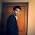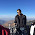# How to Read a Box Plot

Box Plot

A box plot shows the distribution of data. It is useful in visualizing skewness in data.

How to Read a Box Plot

Interpretation

Normal Distribution or Symmetric Distribution : If a box plot has equal proportions around the median, we can say distribution is symmetric or normal.

Positively Skewed : For a distribution that is positively skewed, the box plot will show the median closer to the lower or bottom quartile.
A distribution is considered "Positively Skewed" when mean > median. It means the data constitute higher frequency of high valued scores.
Negatively Skewed : For a distribution that is negatively skewed, the box plot will show the median closer to the upper or top quartile.
A distribution is considered "Negatively Skewed" when mean < median. It means the data constitute higher frequency of low valued scores.
Outlier : If a value is higher than the 1.5*IQR above the upper quartile (Q3), the value will be considered as outlier. Similarly, if a value is lower than the 1.5*IQR below the lower quartile (Q1), the value will be considered as outlier.

Note: IQR is interquartile range. It measures dispersion or variation. IQR = Q3 -Q1.

Better Alternative to Histogram

It is usually better for comparing distributions between several groups or data sets.Related PostsDeepanshu founded ListenData with a simple objective - Make analytics easy to understand and follow. He has over 8 years of experience in data science. During his tenure, he has worked with global clients in various domains like Banking, Insurance, Telecom and Human Resource.

7 Responses to "How to Read a Box Plot"
1.1.http://www.listendata.com/2014/10/cluster-analysis-using-sas.html

2.Thanks for sharing above information. This really helped me indeed.
I believe box plot is the best way to identify outliers in our linear regression model.
To create box plot I mention plot in options in proc univariate SAS, do you know any other procedure or option by which we can create box plot and to make it more presentable.

1.Glad you found it useful. Yes, you can customize box plot by using PROC BOXPLOT procedure.

3.I am finding a hard time with the fences though, because lower fence to Q1 and upper fence to Q3 don't look equal in general. Any help on clarifying my confusion will be greatly appreciated

4.could you please explain me how to judge the kurtosis value positive , negative or zero based on box plot examples?It has been asked in Predictive modeling using SAS e-miner global certification examination.

5.Thanks but I think this can confuse
"It means the data constitute higher frequency of high valued scores"

The use of the word frequency will imply to some, that there are more data points above the median. That isn't the case.
What it is telling me that the range of scores below the median is smaller that the range of scores above the median and that's why the mean can be greater than the median a nd present positive skew

Next → ← Prev
Love this Post? Spread the Word!
Share